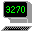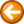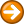If - branch based on the results of a comparisonif condition [AND|OR condition] then statement

if condition [AND|OR condition] then

statement(s)

end if

if condition [AND|OR condition] then

statement(s)

else

statement(s)

end if

if condition [AND|OR condition] then

statement(s)

elseIf condition [AND|OR condition] then

statement(s)

else

statement(s)

end if

Where:

 condition is an expression that evaluates to true or false. See the "Comparison Operators" in Script Operators for list of operators you can use in your If statements.
 AND|OR are keywords used to combine conditional statements in the same IF command. The AND and OR keywords are not case sensitive. Use AND when all of two or more conditions must be true.  Use OR when one of two or more conditions must be true. Multiple AND and OR keywords may be used in a single IF statement.
 statement(s) is a script statement or statements to be executed if the condition is true.  If there are multiple statements, each statement must be on a separate line.

The If command tests a condition or conditions and conditionally processes one or more statements based on the result.

Nested If commands are not supported.

Examples

if \$name EQ "John" then type("Doe")

if \$SCREEN(1,1,1,4) = "John" then

\$user = "John"

else

\$user = "Other"

end if

if \$name = "John" then goto ProcessName:

if \$name LIKE "J*" then goto ProceesJ:

if \$number GT 100 then goto ProcessLarge:

if \$a = 0 and \$b = 0 then goto End:

if \$a = 0 OR \$b = 0 then goto End:

if \$a = 0 and \$b = 0 or \$c = 0 then goto End: